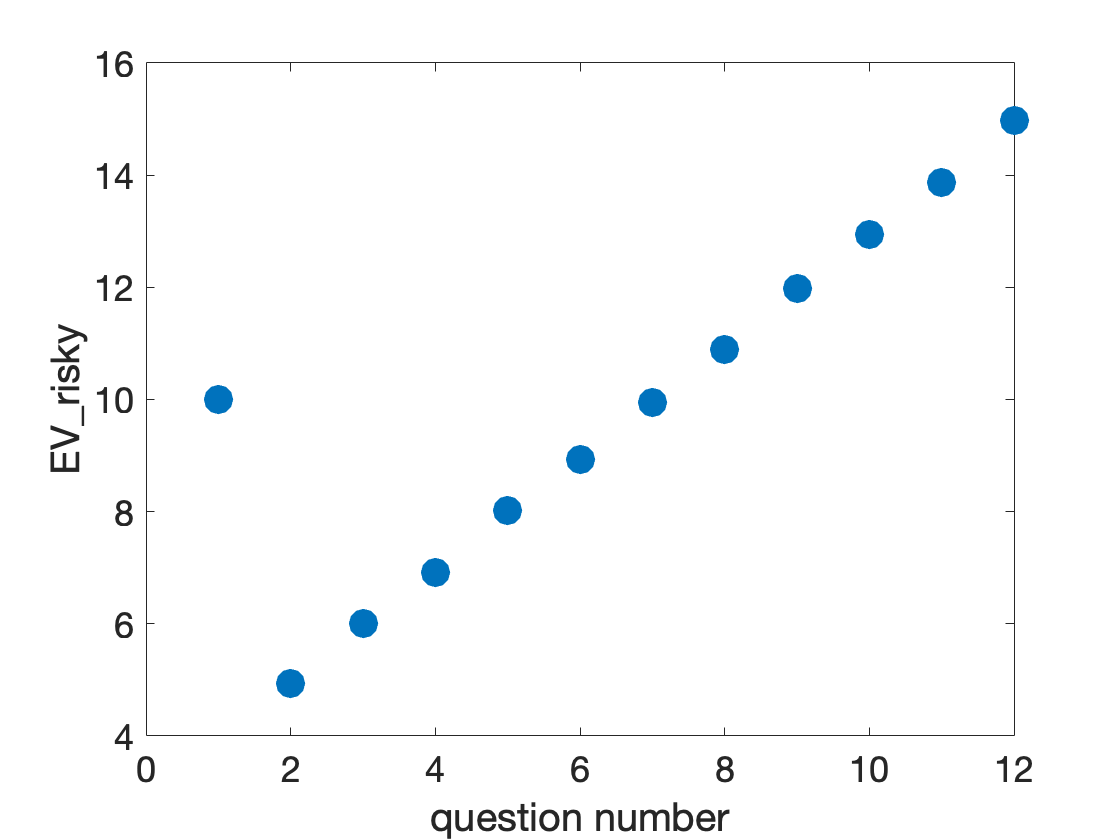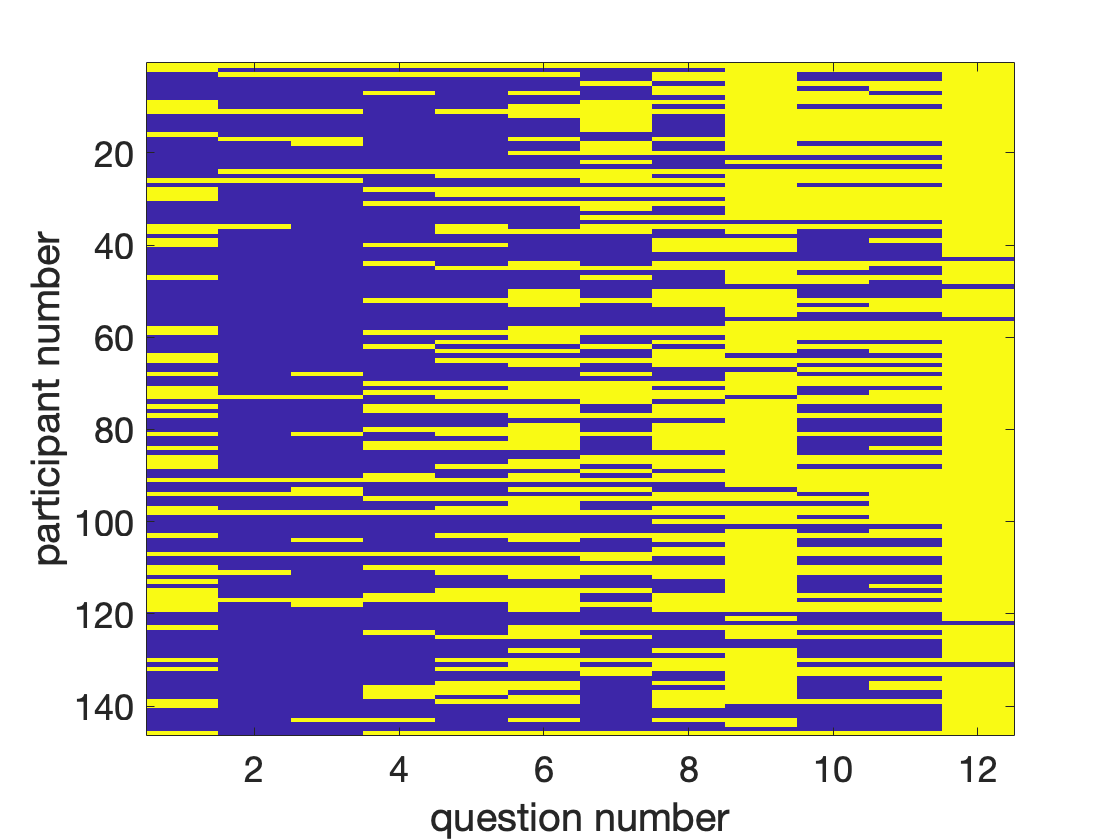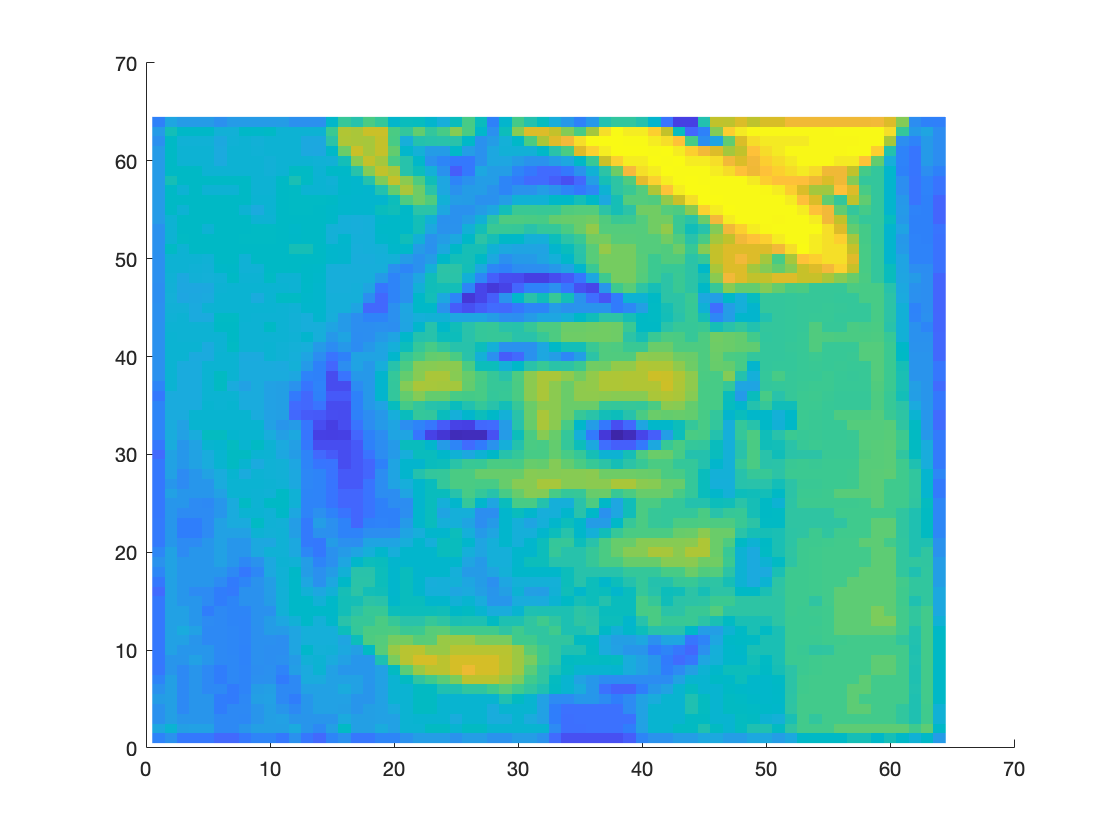# NSCS 344, Week 7

Last time we introduced Expected Value theory and modeled how it might behave on the survey. Today you are going to explore your own data (assuming you did the survey) as well as data from other students who have taken this class.
riskyChoiceData_2020.mat
from D2L.
Once you've downloaded the data make sure you move it into the directory you are writing your scripts in for this week (i.e. the Week_07 directory in your NSCS folder on Dropbox).
Then, from a script, you can load the data like this
clear
This loads a bunch of variables into Matlab. Let's take a look at what we've got using the "whos" command
whos
Name Size Bytes Class Attributes BR 1x146 1168 double P 1x12 96 double QUS 1x12 1818 cell V 1x12 96 double rsk 146x12 14016 double
We've got 5 variables here
• QUS - a "cell" array of strings with the text of each question in the survey
• P - a vector of 12 probabilities for the risky option in each question
• V - a vector of the 12 winning amounts for the risky option in each question
• rsk - a matrix of people's responses to each of the 12 questions. 1 denotes a risky choice, 0 denotes a safe choice.
• BR - a vector containing the blink rates of all the people in the data set in blinks per minute
Note: Because I am making this example before this year's class completed the survey, I have fewer participants in my data set (146) than you will have.

## The questions, QUS

We can explore these variables more in the Command Window. Let's start with the text of the questions, which we can get by typing ...
QUS'
ans = 12×1 cell
'50% chance to win \$20'
'25% chance to win \$19.74'
'26% chance to win \$23.12'
'58% chance to win \$11.93'
'50% chance to win \$16.04'
'62% chance to win \$14.41'
'28% chance to win \$35.52'
'58% chance to win \$18.79'
'71% chance to win \$16.88'
'34% chance to win \$38.04'
Each line here corresponds to the text of the risky option in each question (e.g. question 1 had a risky option of 50% chance to win \$20). Remember that the safe option in this survey was always \$10 for sure.
If you want to look at the text of just one question, say question 4, you can type
QUS{4}
ans = '58% chance to win \$11.93'
Note that you have to use curly brackets { and } with cell arrays.
We're not actually going to do any more with QUS - it's mainly in here just to reorient you to the survey and in case you want to refer to the questions themselves.

## Probabilities and values

Next let's take a closer look at P and V ...
P
P = 1×12
0.5000 0.2500 0.2600 0.5800 0.5000 0.6200 0.2800 0.5800 0.7100 0.3400 0.3600 0.7500
V
V = 1×12
20.0000 19.7400 23.1200 11.9300 16.0400 14.4100 35.5200 18.7900 16.8800 38.0400 38.5100 19.9700
P is a vector of 12 numbers telling you the probability of winning in risky option for each question. So
P(4)
ans = 0.5800
consistent with a 58% chance on question four.
V is a vector of 12 numbers telling you the value of winning for the risky option. So
V(4)
ans = 11.9300
consistent with a winning payout of \$11.93 on question 4.
Using P and V we can compute the Expected Value of the risky option for each question. One way to do this is directly with a for loop
for i = 1:length(P)
EV_risky(i) = P(i) * V(i);
end
Or you could do it using element-wise multiplication
EV_risky = P.*V;
Or you could reuse your EVtheory_survey function from last week. If you want to do this be sure to copy and paste the function into your directory ...
for i = 1:length(P)
[EV_safe(i), EV_risky(i), choice(i)] = EVtheory_survey(10, P(i), V(i));
end
This approach also gives you the choice that pure EV theory would make for "free."
Let's see what those Expected Values are
EV_risky'
ans = 12×1
10.0000 4.9350 6.0112 6.9194 8.0200 8.9342 9.9456 10.8982 11.9848 12.9336
Note: I used the transpose here to transform EV_risky from a row vector into a column vector for easier viewing.
To get an even better idea about EV_risky let's plot it as a function of question number
clf;
plot(EV_risky, '.', 'markersize', 50)
xlabel('question number')
ylabel('EV_risky', 'interpreter', 'none')
set(gca, 'fontsize', 18)This reveals the design of the experiment. I started with an "easy" question
QUS{1}
ans = '50% chance to win \$20'
Which has simple numbers and where the Expected Value is just 10. Then I used more complicated questions like
QUS{2}
ans = '25% chance to win \$19.74'
These questions were designed such that EV_risky would go from about 5 to about 15 in steps of 1. Hence the (very near) linear increase in EV_risky with question number from question 2 to 12 in the plot.
Why did I design the experiment like this? Well, I wanted a range of differences between EV_risky and EV_safe (which remember is always 10). This will allow us to compute the choice curve for people just like we computed the choice curve for the model last week. However, before we can compute the choice curve, we need to take a closer look at the choice data ...

## The choices

The actual choices for each person on each question are in the matrix rsk. For me this matrix has size 146 x 12, meaning that there are 146 rows (1 per subject) and 12 columns (1 per question).
Note you will have more subjects in your data set than I do because it will also include this year's participants.
So if I look at rsk(103, 10) I get the choice of subject number 103 on question 10
rsk(103,10)
ans = 1
A value of 1 indicates that this person chose the risky option on this question.
If we want to look at all the choices made by participant 103 we can write
rsk(103,:)
ans = 1×12
1 0 0 0 1 1 1 1 1 1 1 1
which gives us a row vector of length 12.
Note the special use of the ":" here it's saying "take the whole of row 103."
If we want to look at an entire column (i.e. all the responses to one question, say question 5) we can write
rsk(:,5)
ans = 146×1
1 0 1 0 0 0 0 0 0 0
Which gives us a long column vector containing one entry per participant.
Finally, we can view the whole matrix by just typing in
rsk
rsk = 146×12
1 1 1 1 1 1 1 1 1 1 1 1 1 0 0 0 0 0 0 0 1 1 1 1 0 1 1 1 1 1 0 1 1 0 0 1 0 0 0 0 0 0 0 1 1 0 0 1 0 0 0 0 0 0 1 0 1 1 1 1 0 0 0 0 0 0 0 1 1 0 1 1 0 0 0 1 0 1 0 1 1 1 0 1 0 0 0 0 0 0 0 0 1 1 1 1 1 0 0 0 0 0 1 1 1 1 1 1 1 0 0 0 0 1 1 0 1 0 0 1
However, as nice as it is to look at individual raw data points (and I always recommend doing this if you have data of your own - for example in your project for this class) it would be nice if we could visualize things a bit better. One way to do this is to make an image of the matrix like this ...
imagesc(rsk)
xlabel('question number')
ylabel('participant number')
set(gca, 'fontsize', 18)In this plot we get to see the whole matrix at once. Question number is on the x-axis and subject number is on the y-axis. 1s (risky choices) are yellow and 0s (safe choices) are blue. There's definitely some structure here - there's more yellow on the right of the plot and more blue towards the left. We'll explore this in more detail in a moment, but first a ghost story ...

## The Matlab ghost

It turns out that Matlab is haunted by the ghost of a child. You can see this for yourself by typing imagesc without any input ...
clf;
imagescIn fact it's even spookier than that and there are actually all sorts of creepy dogs, people, and objects hidden in this image at different scales ... here's a gif I made of some of them ...